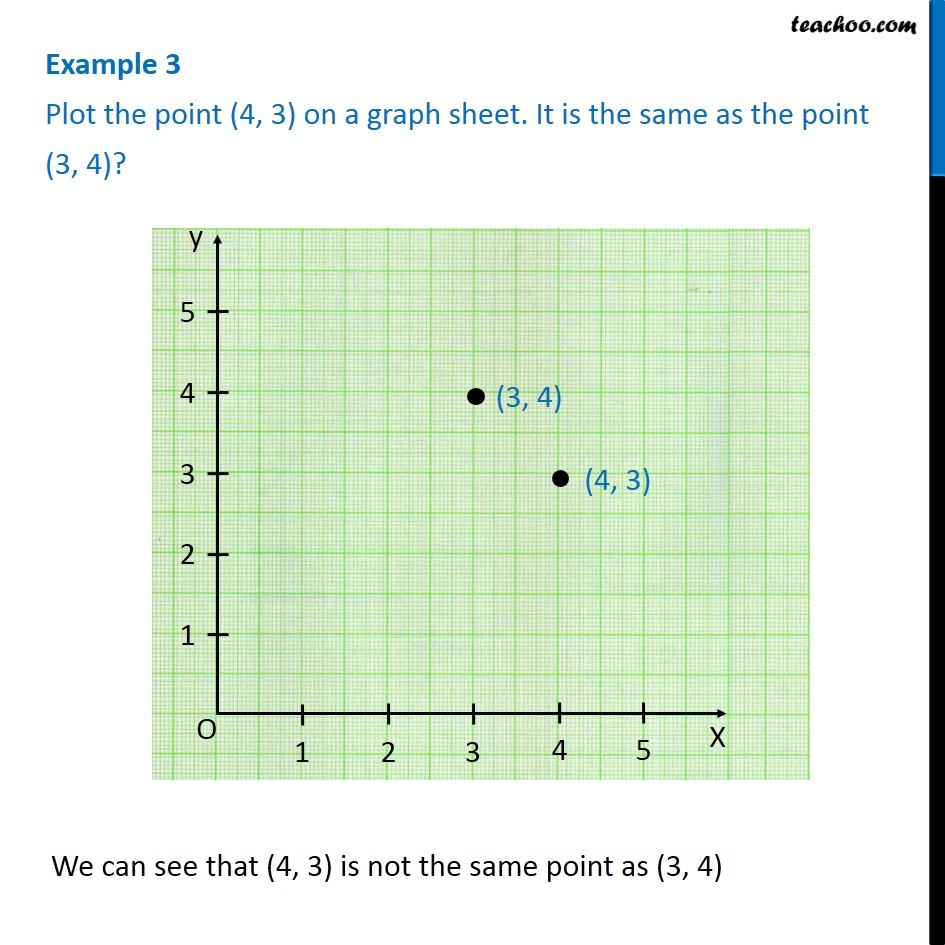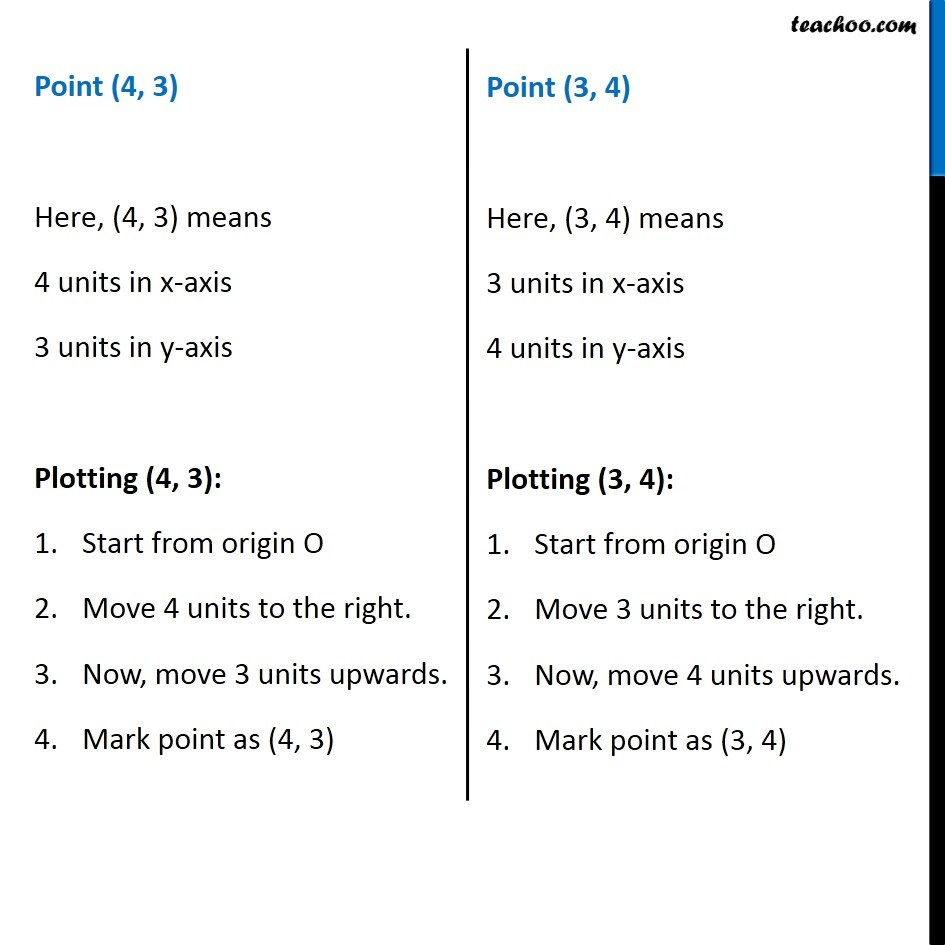Examples

Chapter 13 Class 8 Introduction to Graphs
Serial order wiseLearn in your speed, with individual attention - Teachoo Maths 1-on-1 Class

### Transcript

Question 1 Plot the point (4, 3) on a graph sheet. It is the same as the point (3, 4)? We can see that (4, 3) is not the same point as (3, 4) Point (4, 3) Here, (4, 3) means 4 units in x-axis 3 units in y-axis Plotting (4, 3): Start from origin O Move 4 units to the right. Now, move 3 units upwards. Mark point as (4, 3) Point (3, 4) Here, (3, 4) means 3 units in x-axis 4 units in y-axis Plotting (3, 4): Start from origin O Move 3 units to the right. Now, move 4 units upwards. Mark point as (3, 4)